Courses

# RD Sharma Solutions Ex-24.1, (Part - 1), Measures Of Central Tendency, Class 9, Maths Class 9 Notes | EduRev

## Class 9 : RD Sharma Solutions Ex-24.1, (Part - 1), Measures Of Central Tendency, Class 9, Maths Class 9 Notes | EduRev

The document RD Sharma Solutions Ex-24.1, (Part - 1), Measures Of Central Tendency, Class 9, Maths Class 9 Notes | EduRev is a part of the Class 9 Course RD Sharma Solutions for Class 9 Mathematics.
All you need of Class 9 at this link: Class 9

Q.1: If the heights of 5 persons are 140 cm, 150 cm, 152 cm, 158 cm and 161 cm respectively. Find the mean height.

SOLUTION :

Given : the heights of 5 persons are 140 cm , 150 cm , 152 cm , 158 cm and 161 cm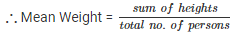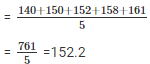Q 2 . Find the mean of 994 , 996 , 998 , 1000 , 1002.

SOLUTION :

Given :

Numbers are 994 , 996 , 998 , 1000 , 1002.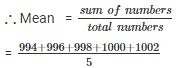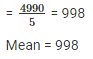Q 3 . Find the mean of first five natural numbers.

SOLUTION :

The first five odd numbers are 1 , 2 , 3 , 4 , 5.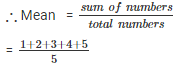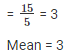Q 4 . Find the mean of all factors of 10.

SOLUTION :

All factors of 6 are 1 , 2 , 5 , 10.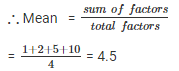Mean = 4.5

Q 5 . Find the mean of first ten even natural numbers.

SOLUTION :

The first five even natural numbers are 2 , 4 , 6 , 8 , 10 , 12 , 14 , 16 , 18 , 20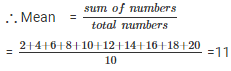Mean = 11

Q 6 . Find the mean of x , x + 2 , x + 4 , x + 6 , x + 8.

SOLUTION :

Numbers are x , x + 2 , x + 4 , x + 6 , x + 8.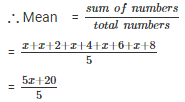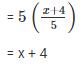Q 7. Find the mean of first five multiples of 3.

SOLUTION :

First five multiples of 3 are 3 , 6 , 9 , 12 , 15.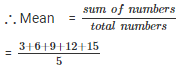= 9

Mean = 9

Q 8 . Following are the weights of 10 new born babies in a hospital on a particular day  : 3.4 , 3 .6 , 4.2 , 4.5 , 3.9 , 4.1 , 3.8 , 4.5 , 4.4 , 3.6 (in kg). Find the mean.

SOLUTION : The weights (in kg) of 10 new born  babies are : 3.4 , 3 .6 , 4.2 , 4.5 , 3.9 , 4.1 , 3.8 , 4.5 , 4.4 , 3.6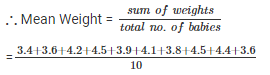= 4 kg

Q 9 . The percentage marks obtained by students of a class in mathematics are as follows: 64 , 36 , 47 , 23 , 0 , 19 , 81 , 93 , 72 , 35 , 3 , 1 .Find their mean.

SOLUTION :

The percentage marks obtained by students are 64 , 36 , 47 , 23 , 0 , 19 , 81 , 93 , 72 , 35 , 3 , 1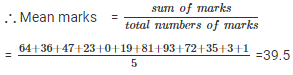Mean Marks = 39.5

Q 10. The numbers of children in 10 families of a locality  are 2 , 4 , 3 , 4 , 2 , 3 , 5 , 1 , 1 , 5 . Find the number of children per family.

SOLUTION :

The numbers of children  in 10 families  are : 2 , 4 , 3 , 4 , 2 , 3 , 5 , 1 , 1 , 5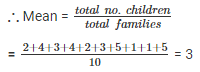Q 11 . If M is the mean of x1,x2,x3,x4,xand x6 , Prove that

(x1−M)+(x2−M)+(x3−M)+(x4−M)+(x5−M)+(x6−M) = 0.

SOLUTION :

Let M be the mean of x1,x2,x3,x4,xand x6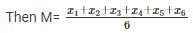= x1+x2+x3+x4+x5+x6 = 6M

To Prove :- (x1−M)+(x2−M)+(x3−M)+(x4−M)+(x5−M)+(x6−M) = 0.

Proof :- L . H . S

= (x1−M)+(x2−M)+(x3−M)+(x4−M)+(x5−M)+(x6−M)

= (x1+x2+x3+x4+x5+x6)−(M+M+M+M+M+M)

= 6M – 6M

= 0

= R . H . S

Q 12 . Duration of sunshine(in hours) in  Amritsar  for first  10 days of August  1997 as reported by the Meterological Department  are given as  follows : 9.6 , 5.2 , 3.5 , 1.5 , 1.6 , 2.4 , 2.6 , 8.4 , 10.3 , 10.9

1. Find the mean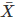2.Verify that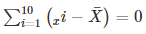SOLUTION :

Duration of sunshine (in hours ) for 10 days are =9.6 , 5.2 , 3.5 , 1.5 , 1.6 , 2.4 , 2.6 , 8.4 , 10.3 , 10.9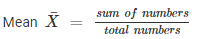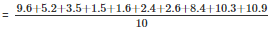= 56/10 = 5.6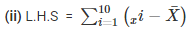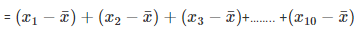= (9.6−5.6)+(5.2−5.6)+(3.5−5.6)+(1.5−5.6)+(1.6−5.6)+(2.4−5.6)+(2.6−5.6)+(8.4−5.6)+(10.3−5.6)+(10.9−5.6)

= 4 – 0.4 – 2.1 – 4.1 – 4 – 3.2 – 3 + 2.8 + 4.7 + 5.3

= 16.8 – 16.8 = 0

= R.H.S

Offer running on EduRev: Apply code STAYHOME200 to get INR 200 off on our premium plan EduRev Infinity!

91 docs

,

,

,

,

,

,

,

,

,

,

,

,

,

,

,

,

,

,

,

,

,

,

,

,

,

,

,

,

,

,

,

,

,

;